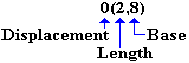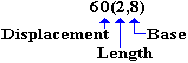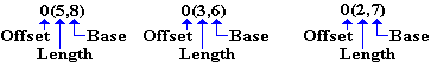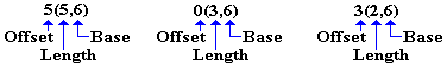Type RS Instruction Format

This is a four–byte instruction of the form OP R1,R3,D2(B2).

 Type Bytes Operands 1 2 3 4 RS 4 R1,R3,D2(B2) OP R1 R3 B2 D2 D2D2

The first byte contains the 8–bit instruction code.

The second byte contains two 4–bit fields, each of which encodes a register number.  Some RS format instructions use only one register, here R3 is set to 0.  This instruction format follows the IBM architecture standard that “0” is taken as no register, rather than register R0.

The third and fourth byte contain a 4–bit register number and 12–bit displacement, used to specify the memory address for the operand in storage.  Recall that each label in the assembly language program references an address.

Any address in the format of base register and displacement will appear in the form.

 B D1 D2 D3

B is the hexadecimal digit representing the base register.

The three hexadecimal digits D1 D2 D3 form the 12–bit displacement, which is to be interpreted as a non–negative integer in the range from 0 through 4095, inclusive.

As an example of the type, we consider the BXH instruction with opcode X‘86’.

A standard use of the instruction would be as follows.

BXH R6,R8,L10LOOP

It is important to remember that the above could be written in source code in this form.

LA  R4,L10LOOP     ADDRESS OF LABEL L10LOOP INTO R4
BXH R6,R8,0(4)     BRANCH TARGET ADDRESS IN R4.

One might have an instruction of the following form as well.

BXH R6,R8,12(4)    BRANCH TARGET ADDRESS IS DISPLACED
12 (X‘C’) FROM ADDRESS IN R4.

RX (Register–Indexed Storage) Format

This is a four–byte instruction of the form OP R1,D2(X2,B2).

 Type Bytes Operands 1 2 3 4 RX 4 R1,D2(X2,B2) OP R1 X2 B2 D2 D2D2

The first byte contains the 8–bit instruction code.

The second byte contains two 4–bit fields, each of which encodes a register number.  The first hexadecimal digit, denoted R1, identifies the register to be used as either the source or destination for the data.  The second hexadecimal digit, denoted X2, identifies the register to be used as the index.  If the value is 0, indexed addressing is not used.

The third and fourth bytes contain a standard address in base/displacement format.

As an examples of this type, we consider the two following instructions:
L          Load Fullword                        Opcode is X‘58’
A          Add Fullword             Opcode is X‘5A’

We consider a number of examples based on the following data declarations.  Note that the data are defined in consecutive fullwords in memory, so that fixed offset addressing can be employed.  Each fullword has a length of four bytes.

DAT1      DC F‘1111’

DAT2      DC F‘2222’     AT ADDRESS (DAT1 + 4)

DAT3      DC F‘3333’     AT ADDRESS (DAT2 + 4) OR (DAT1 + 8)

A standard code block might appear as follows.

L R5,DAT1

A R5,DAT2

A R5,DAT3      NOW HAVE THE SUM.

One variant of this code might be the following.  See page 92 of R_17.

LA R3,DAT1      GET ADDRESS INTO R3

L  R5,0(,3)     LOAD DAT1 INTO R5

Note the leading comma in the construct (,3), which is of the form (Index, Base).  This indicates that no index register is being used, but that R3 is being used as a base register.  It is equivalent to the construct (0,3), which might be preferred.

Here is another variant of the above code.

LA R3,DAT1      GET ADDRESS INTO R3

LA R8,4         VALUE 4 INTO REGISTER 8

LA R9,8         VALUE 8 INTO REGISTER 9

L  R5,0(0,3)    LOAD DAT1 INTO R5

Explicit Base Addressing for Character Instructions

We now discuss a number of ways in which the operand addresses for character instructions may be presented in the source code.  One should note that each of these source code representations will give rise to object code that appears almost identical.  These examples are taken from Peter Abel [R_02, pages 271 – 273].

Assume that general–purpose register 4 is being used as the base register, as assigned at
the beginning of the CSECT.  Assume also that the following statements hold.

1.   General purpose register 4 contains the value X‘8002’.

2.   The label PRINT represents an address represented in base/offset form as 401A; that
is it is at offset X‘01A’ from the value stored in the base register, which is R4.
The address then is X‘8002’ + X‘01A’ = X‘801C’.

3.   Given that the decimal number 60 is represented in hexadecimal as X‘3C’,
the address PRINT+60 must then be at offset X‘01A’ + X‘3C’ = X‘56’ from
the address in the base register.  X‘A’ + X‘C’, in decimal, is 10 + 12 = 16 + 6.

Note that this gives the address of PRINT+60 as X‘8002’ + X‘056’ = X‘8058’,
which is the same as X‘801C’ + X‘03C’.  The sum X‘C’ + X‘C’, in decimal, is
represented as 12 + 12 = 24 = 16 + 8.

4.   The label ASTERS is associated with an offset of X‘09F’ from the value in the
base register; thus it is located at address X‘80A1’.  This label references a storage
of two asterisks.  As a decimal value, the offset is 159.

5.   That only two characters are to be moved by the MVC instruction examples to be
discussed.  Since the length of the move destination is greater than 2, and since the
length of the destination is the default for the number of characters to be moved, this
implies that the number of characters to be moved must be stated explicitly.

The first example to be considered has the simplest appearance.  It is as follows:

MVC PRINT+60(2),ASTERS

The operands here are of the form Destination(Length),Source.
The destination is the address PRINT+60.  The length (number of characters
to move) is 2.  This will be encoded in the length byte as X‘01’, as the length
byte stores one less than the length.  The source is the address ASTERS.

As the MVC instruction is encoded with opcode X‘D2’, the object code here is as follows:

 Type Bytes Operands 1 2 3 4 5 6 SS(1) 6 D1(L,B1),D2(B2) OP L B1 D1 D1D1 B2 D2 D2D2 D2 01 40 56 40 9F

The next few examples are given to remind the reader of other ways to encode
what is essentially the same instruction.

These examples are based on the true nature of the source code for a MVC instruction, which is MVC D1(L,B1),D2(B2).  In this format, we have the following.

1.   The destination address is given by displacement D1 from the address stored in
the base register indicated by B1.

2.   The number of characters to move is denoted by L.

3.   The source address is given by displacement D2 from the address stored in
the base register indicated by B2.

The second example uses an explicit base and displacement representation of the destination address, with general–purpose register 8 serving as the explicit base register.

LA  R8,PRINT+60     GET ADDRESS PRINT+60 INTO R8

MVC 0(2,8),ASTERS   MOVE THE CHARACTERS

Note the structure in the destination part of the source code, which is 0(2,8).The displacement is 0 from the address X‘8058’, which is stored in R8.  The object code is:

 Type Bytes Operands 1 2 3 4 5 6 SS(1) 6 D1(L,B1),D2(B2) OP L B1 D1 D1D1 B2 D2 D2D2 D2 01 80 00 40 9F

The instruction could have been written as MVC 0(2,8),159(4), as the label
ASTERS is found at offset 159 (decimal) from the address in register 4.

The third example uses an explicit base and displacement representation of the destination address, with general–purpose register 8 serving as the explicit base register.

LA  R8,PRINT        GET ADDRESS PRINT INTO R8

MVC 60(2,8),ASTERS  SPECIFY A DISPLACEMENT

Note the structure in the destination part of the source code, which is 60(2,8).The displacement is 60 from the address X‘801C’, stored in R8.  The object code is:

 Type Bytes Operands 1 2 3 4 5 6 SS(1) 6 D1(L,B1),D2(B2) OP L B1 D1 D1D1 B2 D2 D2D2 D2 01 80 3C 40 9F

The instruction could have been written as MVC 60(2,8),159(4), as the label
ASTERS is found at offset 159 (decimal) from the address in register 4.

Explicit Base Addressing for Packed Decimal Instructions

We now discuss a number of ways in which the operand addresses for character instructions may be presented in the source code.  One should note that each of these source code representations will give rise to object code that appears almost identical.  These examples are taken from Peter Abel [R_02, pages 273 & 274].

Consider the following source code, taken from Abel.  This is based on a conversion of a weight expressed in kilograms to its equivalent in pounds; assuming 1kg. = 2.2 lb.  Physics students will please ignore the fact that the kilogram measures mass and not weight.

ZAP  POUNDS,KGS     MOVE KGS TO POUNDS

MP   POUNDS,FACTOR  MULTIPLY BY THE FACTOR
SRP  POUNDS,63,5    ROUND TO ONE DECIMAL PLACE

KGS      DC   PL3‘12.53’     LENGTH 3 BYTES

FACTOR   DC   PL2‘2.2’       LENGTH 2 BYTES, AT ADDRESSS KGS+3

POUNDS   DS   PL5            LENGTH 5 BYTES, AT ADDRESS KGS+5

The value produced is 12.53·2.2 = 27.566, which is rounded to 27.57.

The instructions we want to examine in some detail are the MP and ZAP, each of which
is a type SS instruction with source code format OP D1(L1,B1),D2(L2,B2).  Each of the two operands in these instructions has a length specifier.

In the first example of the use of explicit base registers, we assign a base register to
represent the address of each of the arguments.  The above code becomes the following:

LA R6,KGS           ADDRESS OF LABEL KGS

LA R8,POUNDS

ZAP 0(5,8),0(3,6)

MP  0(5,8),0(2,7)

SRP 0(5,8),63,5

Each of the arguments in the MP and ZAP have the following form:Recall the definitions of the three labels, seen just above.  We analyze the instructions.

ZAP 0(5,8),0(3,6)  Destination is at offset 0 from the address
stored in R8. The destination has length 5 bytes.

Source is at offset 0 from the address stored
in R6.  The source has length 3 bytes.

MP  0(5,8),0(2,7)  Destination is at offset 0 from the address
stored in R8. The destination has length 5 bytes.

Source is at offset 0 from the address stored
in R7.  The source has length 2 bytes.

But recall the order in which the labels are declared.  The implicit assumption that the labels are in consecutive memory locations will here be made explicit.

KGS      DC   PL3‘12.53’     LENGTH 3 BYTES

FACTOR   DC   PL2‘2.2’       LENGTH 2 BYTES, AT ADDRESSS KGS+3

POUNDS   DS   PL5            LENGTH 5 BYTES, AT ADDRESS KGS+5

In this version of the code, we use the label KGS as the base address and reference all other addresses by displacement from that one.  Here is the code.

LA R6,KGS           ADDRESS OF LABEL KGS

ZAP 5(5,6),0(3,6)

MP  5(5,6),3(2,6)

SRP 5(5,6),63,5

Each of the arguments in the MP and ZAP have the following form:Recall the definitions of the three labels, seen just above.  We analyze the instructions.

ZAP 5(5,6),0(3,6)  Destination is at offset 5 from the address
stored in R6. The destination has length 5 bytes.

Source is at offset 0 from the address stored
in R6.  The source has length 3 bytes.

MP  5(5,6),3(2,6)  Destination is at offset 5 from the address
stored in R6. The destination has length 5 bytes.

Source is at offset 3 from the address stored
in R6.  The source has length 2 bytes.

In other words, the base/displacement 6000 refers to a displacement of 0 from the address stored in register 6, which is being used as an explicit base register for this operation.  As
the address in R6 is that of KGS, this value represents the address KGS.  This is the object code address generated in response to the source code fragment 0(3,6).

The base/displacement 6003 refers to a displacement of 3 from the address stored in register 6, which is being used as an explicit base register for this operation.  As the address in R6 is that of KGS, this value represents the address KGS+3, which is the address FACTOR.  This is the object code address generated in response to the source code fragment 3(2,6).

The base/displacement 6005 refers to a displacement of 5 from the address stored in register 6, which is being used as an explicit base register for this operation.  As the address in R6 is that of KGS, this value represents the address KGS+5, which is the address POUNDS.  This is the object code address generated in response to the source code fragment 5(5,6).

It is worth notice, even at this point, that the use of a single register as the base from which to reference a block of data declarations is quite suggestive of what is done with a DSECT, also called a “Dummy Section”.